Question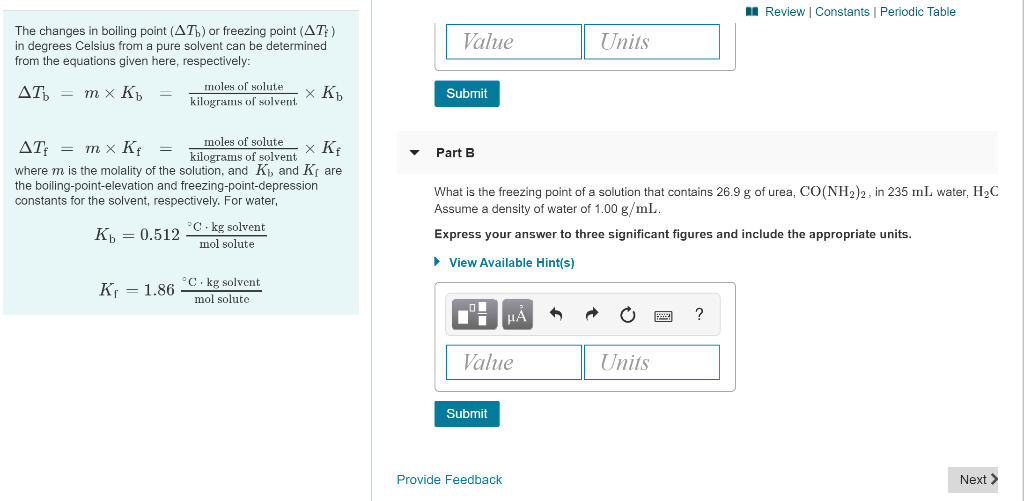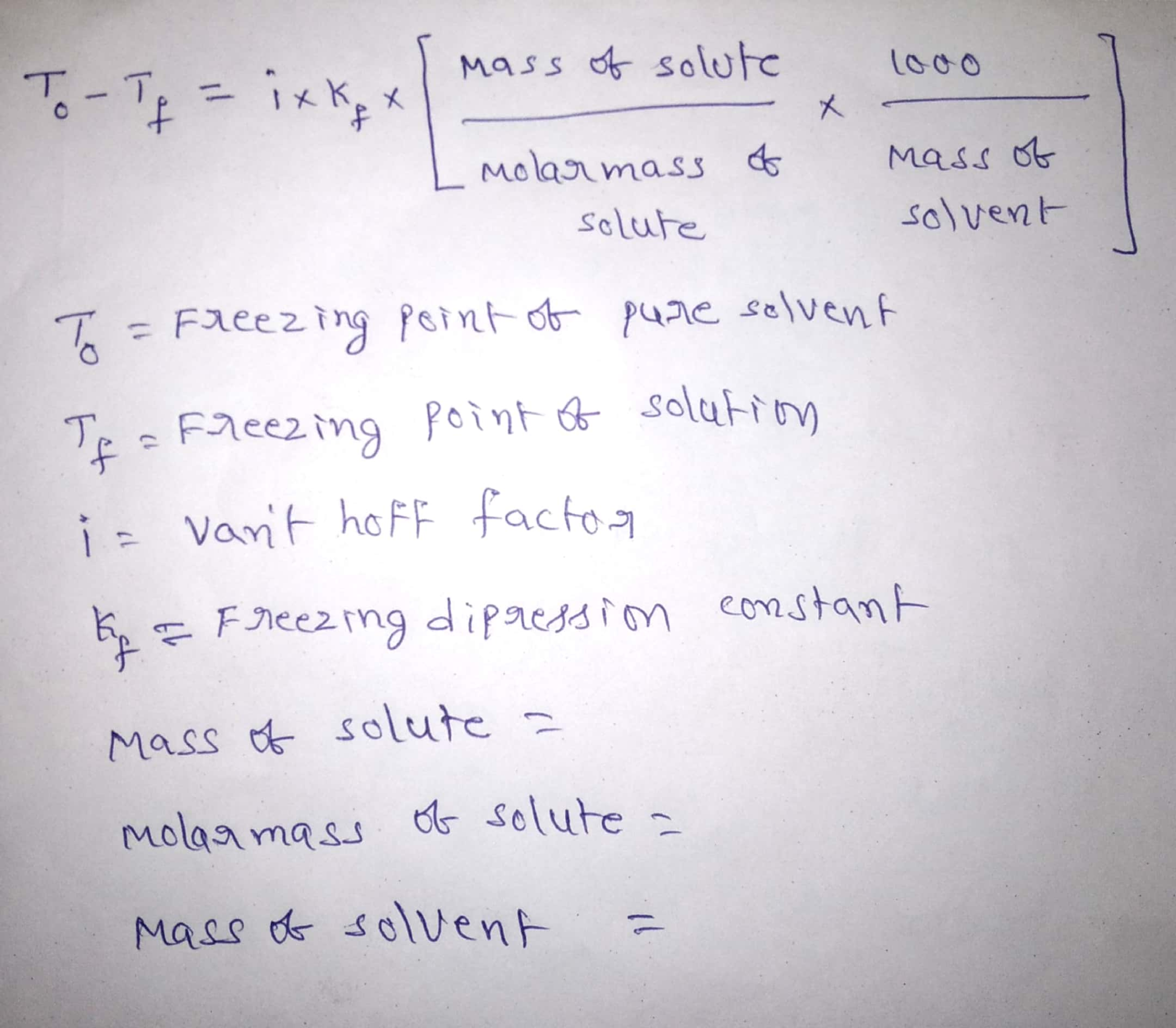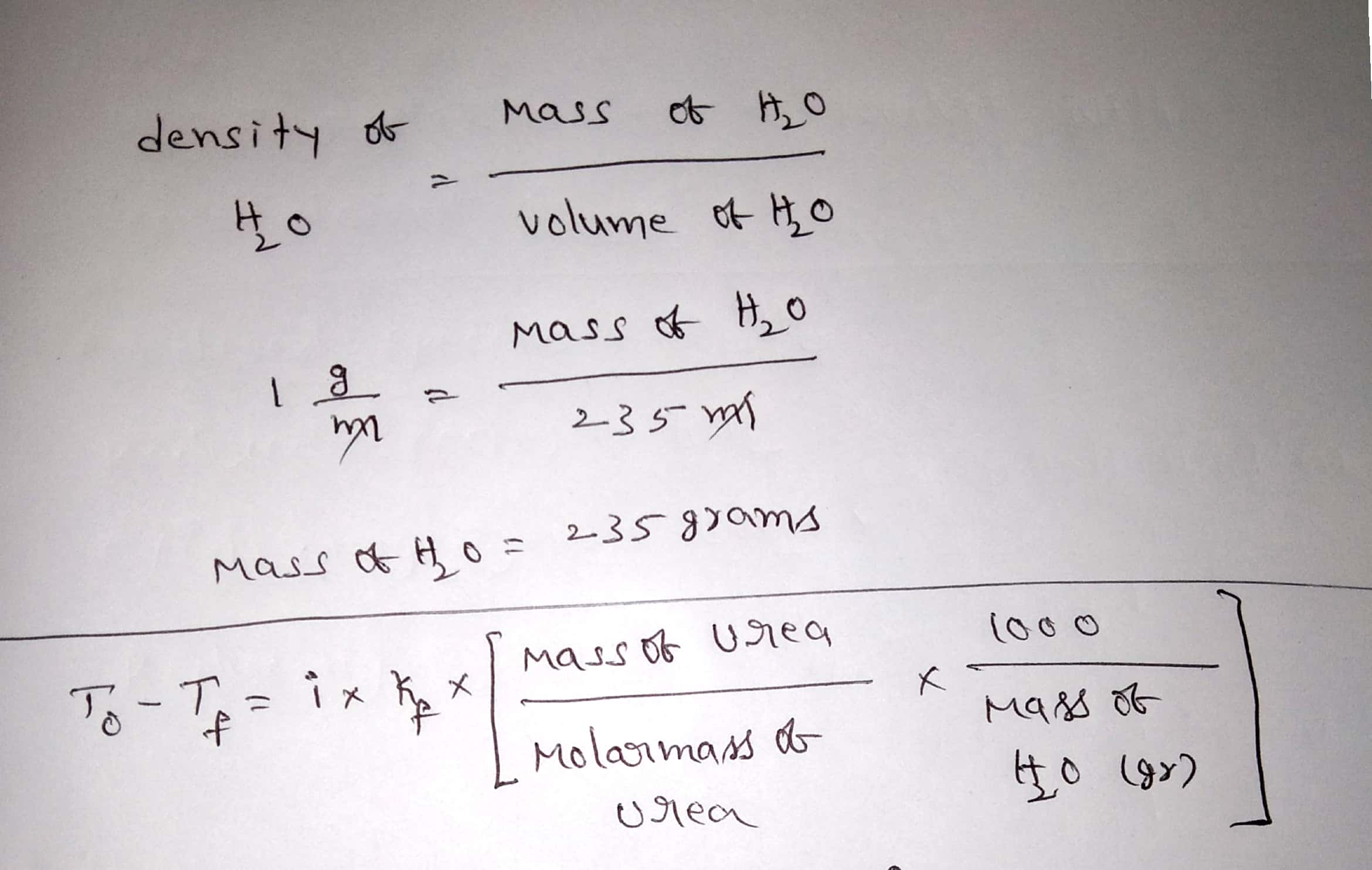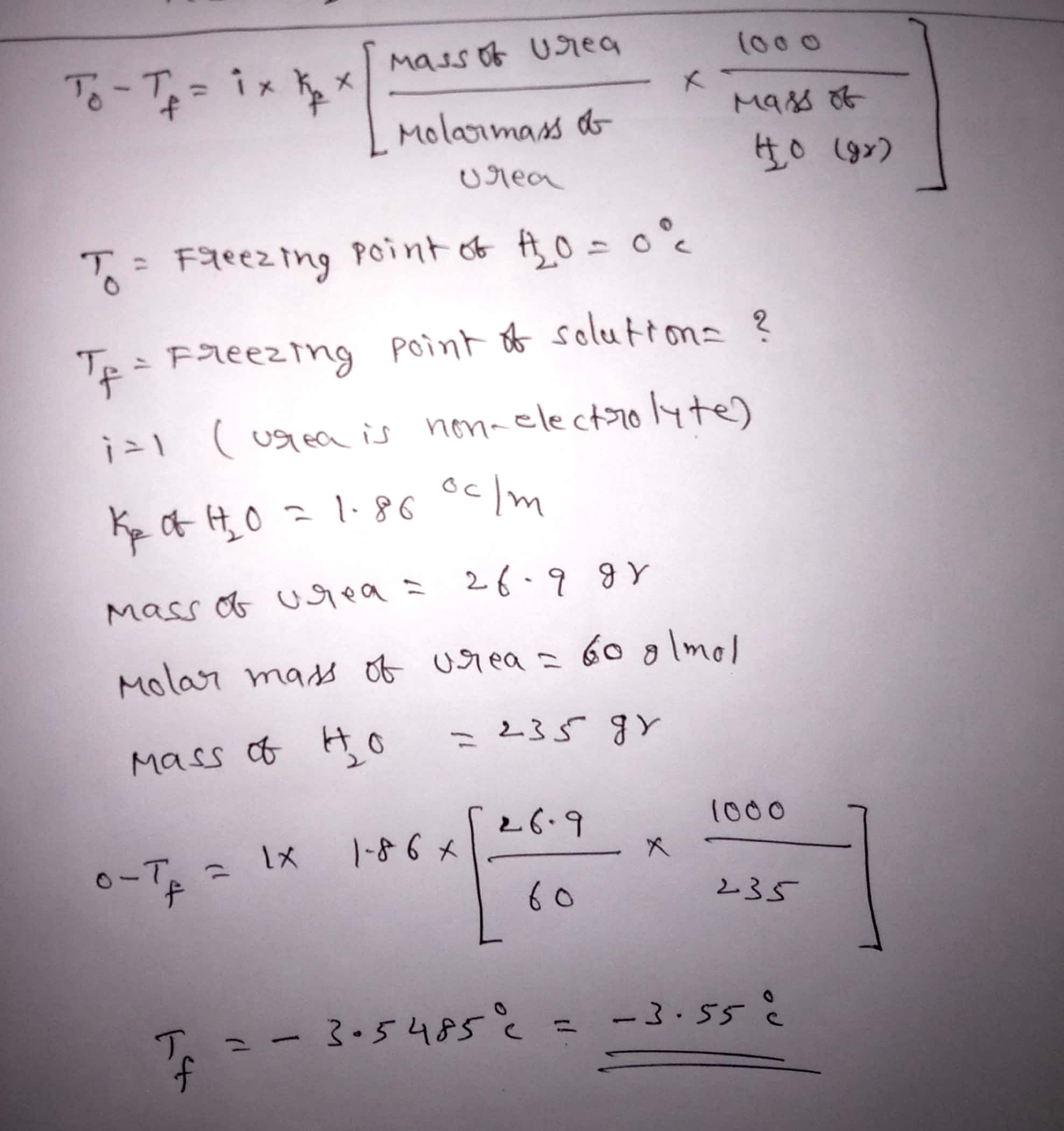#### Earn Coins

Coins can be redeemed for fabulous gifts.

Similar Homework Help Questions
• ### A Review Constants Periodic Table The changes in boiling point (AT) or freezing point (AT) in...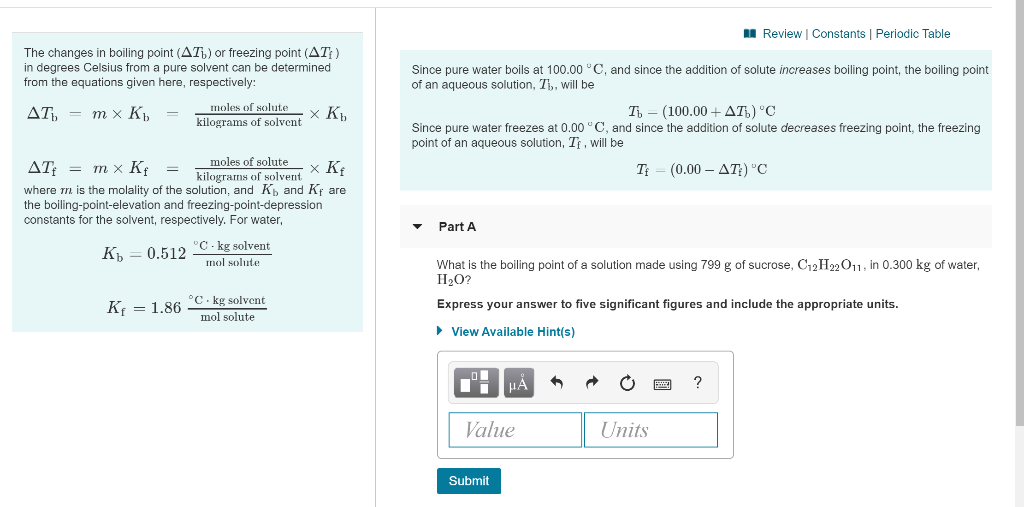A Review Constants Periodic Table The changes in boiling point (AT) or freezing point (AT) in degrees Celsius from a pure solvent can be determined from the equations given here, respectively: AT) = m x K = moles of solute XK K. kilograms of solvent Since pure water boils at 100.00 °C, and since the addition of solute increases boiling point, the boiling point of an aqueous solution, Th, will be T - (100.00+AT) 'C Since pure water freezes at...

• ### Molality, Freezing Point, and Boiling Point 29 of 44 - Part 3 Review Constants Periodic Table...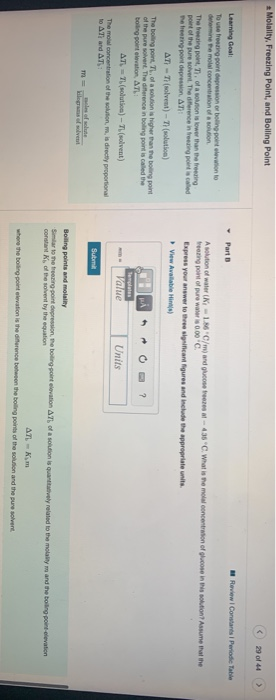Molality, Freezing Point, and Boiling Point 29 of 44 - Part 3 Review Constants Periodic Table Learning Goal Toute ring point depression or boling point elevation to din Pemola concentration of a solution The bring point, T. of a sortion is lower than the freezing point of the pure solvent. The difference in freezing point is called The treening point depression. AT AT - (solvent) - Tolution) The big pont, Th. of a solution is higher than the boting point...

• ### The boiling point of water is 100.00 °C at 1 atmosphere. A student dissolves 12.31 grams...

The boiling point of water is 100.00 °C at 1 atmosphere. A student dissolves 12.31 grams of cobalt(II) acetate, Co(CH3COO)2 (177.0 g/mol), in 287.1 grams of water. Use the table of boiling and freezing point constants to answer the questions below. Solvent Formula Kb (°C/m) Kf (°C/m) Water H2O 0.512 1.86 Ethanol CH3CH2OH 1.22 1.99 Chloroform CHCl3 3.67 Benzene C6H6 2.53 5.12 Diethyl ether CH3CH2OCH2CH3 2.02 The molality of the solution is _____m. The boiling point of the solution is  °____C....

• ### The freezing point of water is 0.00°C at 1 atmosphere. A student dissolves 12.54 grams of chromium(II) acetate, Cr(CH3COO)2 (170.1 g/mol), in 158.2 grams of water. Use the table of boiling and freezin...

The freezing point of water is 0.00°C at 1 atmosphere. A student dissolves 12.54 grams of chromium(II) acetate, Cr(CH3COO)2 (170.1 g/mol), in 158.2 grams of water. Use the table of boiling and freezing point constants to answer the questions below. Solvent Formula Kb (°C/m) Kf (°C/m) Water H2O 0.512 1.86 Ethanol CH3CH2OH 1.22 1.99 Chloroform CHCl3 3.67 Benzene C6H6 2.53 5.12 Diethyl ether CH3CH2OCH2CH3 2.02 The molality of the solution is m. The freezing point of the solution is °C....

• ### part b and c please ( 5 of 15 A Review | Constants Periodic Table Part...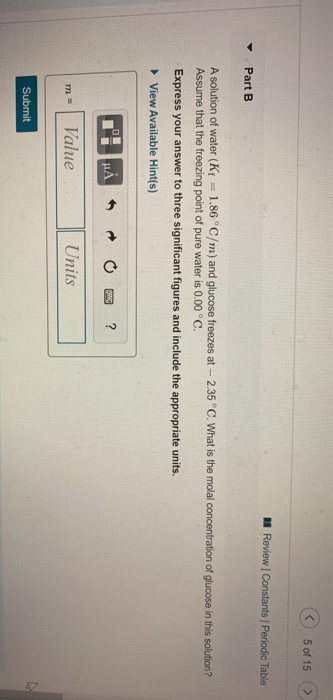part b and c please ( 5 of 15 A Review | Constants Periodic Table Part B A solution of water (Kf = 1.86°C/m) and glucose freezes at -- 2.35 °C. What is the molal concentration of glucose in this solution? Assume that the freezing point of pure water is 0.00 °C. Express your answer to three significant figures and include the appropriate units. View Available Hint(s) T'I HAO ? m= Value Units Submit A Review Constants Periodic Table AT)...

• ### The freezing point of water is 0.00°C at 1 atmosphere. A student dissolves 10.34 grams of...

The freezing point of water is 0.00°C at 1 atmosphere. A student dissolves 10.34 grams of calcium acetate, Ca(CH3COO)2 (158.2 g/mol), in 216.0 grams of water. Use the table of boiling and freezing point constants to answer the questions below. Solvent Formula Kb (°C/m) Kf (°C/m) Water H2O 0.512 1.86 Ethanol CH3CH2OH 1.22 1.99 Chloroform CHCl3 3.67 Benzene C6H6 2.53 5.12 Diethyl ether CH3CH2OCH2CH3 2.02 The molality of the solution is ________m. The freezing point of the solution is ___________...

• ### - Boiling point and Freezing point Elevation Calculate the boiling point and freezing points of water...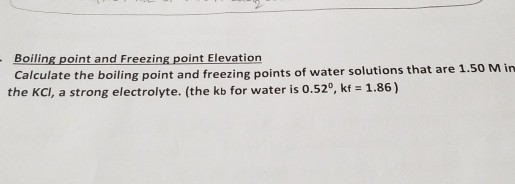- Boiling point and Freezing point Elevation Calculate the boiling point and freezing points of water solutions that are 1.50 Min the KCl, a strong electrolyte. (the kb for water is 0.52", kf = 1.86)

• ### The boiling point of water is 100.00 °C at 1 atmosphere. A student dissolves 12.70 grams of chromium(III) sulfate, Cr2(...

The boiling point of water is 100.00 °C at 1 atmosphere. A student dissolves 12.70 grams of chromium(III) sulfate, Cr2(SO4)3 (392.2 g/mol), in 214.5 grams of water. Use the table of boiling and freezing point constants to answer the questions below. Solvent Formula Kb (°C/m) Kf (°C/m) Water H2O 0.512 1.86 Ethanol CH3CH2OH 1.22 1.99 Chloroform CHCl3 3.67 Benzene C6H6 2.53 5.12 Diethyl ether CH3CH2OCH2CH3 2.02 The molality of the solution is ___?____m. The boiling point of the solution is...

• ### 1h. A certain pure solvent freezes at 39.8°C and has a freezing point depression constant Kf...

1h. A certain pure solvent freezes at 39.8°C and has a freezing point depression constant Kf = 0.777°C/m. What is the predicted freezing point (in °C) of a solution made from this solvent that is (1.90x10^0) m in a non-electrolyte solute? 1i. When (8.23x10^1) g of a non-electrolyte is dissolved in (5.2600x10^2) g of a solvent (with Kb = 0.416°C/m) the boiling point of the solution is 1.50°C higher than the boiling point of the pure solvent. What is the...

• ### 1.The freezing point of benzene is 5.5 °C. What is the molar mass of a nonionizing...

1.The freezing point of benzene is 5.5 °C. What is the molar mass of a nonionizing solute if 1.99 grams of the solute added to 20.62 g of benzene lowers the freezing point to 3.44. The Kf of benzene is -5.12 °C/m. Record your answer as a whole number. 2.Water has a Kf of -1.86 °C/m. Calculate the new freezing point of an aqueous solution made by mixing 38.66 g water and 1.64 g MgCl2. 3.Ethanol has a Kb of...